Chat Now

# Suppose in its income statement for the year ended June 30 2017 The Clorox Company reported the following condensed data

### Suppose in its income statement for the year ended June 30 2017 The Clorox Company reported the following condensed data

Suppose in its income statement for the year ended June 30, 2017, The Clorox Company reported the following condensed data (dollars in millions). NEED THIS BY 11 AM TODAY.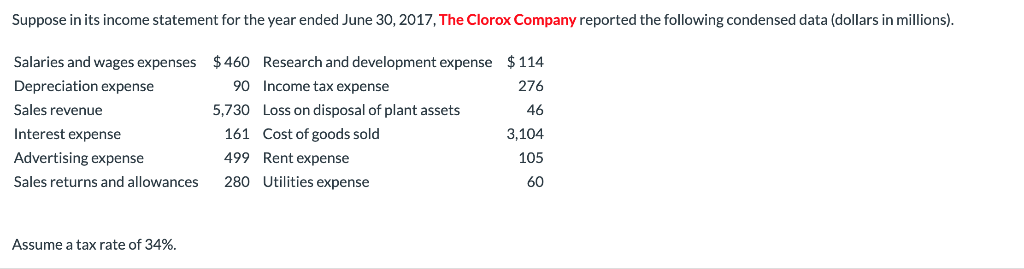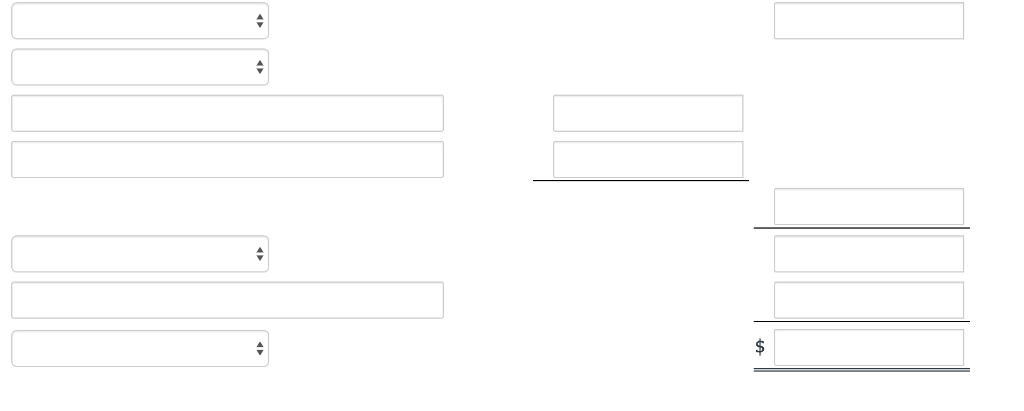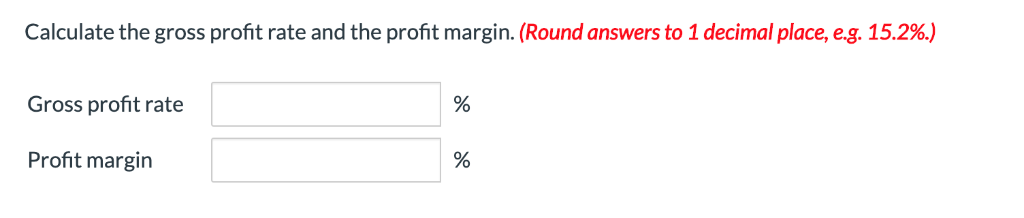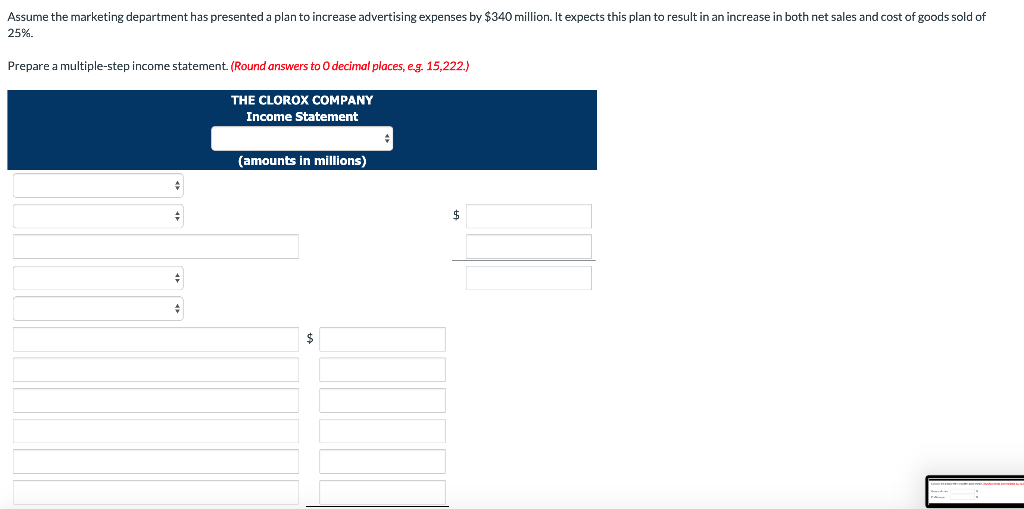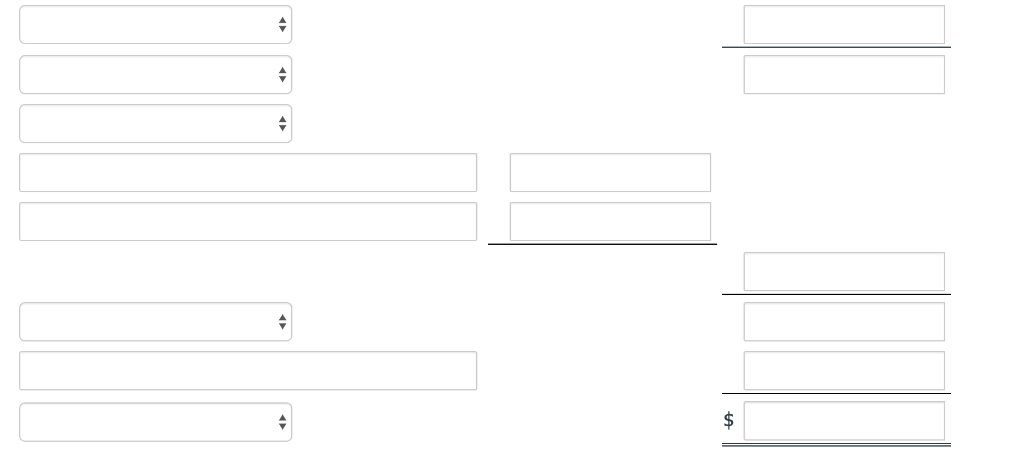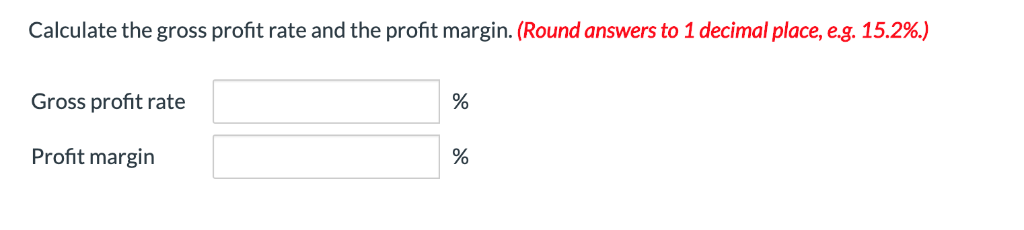Suppose in its income statement for the year ended June 30, 2017, The Clorox Company reported the following condensed data (dollars in millions). Salaries and wages expenses \$460 \$114 Research and development expense Depreciation expense 90 Income tax expense 276 Sales revenue Loss on disposal of plant assets 5,730 46 Interest expense 161 Cost of goods sold 3,104 Advertising expense 499 Rent expense 105 280 Utilities expense Sales returns and allowances 60 Assume a tax rate of 34%. THE CLOROX COMPANY Income Statement (amounts in millions) \$ We were unable to transcribe this imageCalculate the gross profit rate and the profit margin. (Round answers to 1 decimal place, e.g. 15.2%.) Gross profit rate Profit margin % Assume the marketing department has presented a plan to increase advertising expenses by \$340 million. It expects this plan to result in an increase in both net sales and cost of goods sold of 25% Prepare a multiple-step income statement. (Round answers to 0 decimal places, eg. 15,222.) THE CLOROX COMPANY Income Statement (amounts in millions) \$ Calculate the gross profit rate and the profit margin. (Round answers to 1 decimal place, e.g. 15.2%.) Gross profit rate Profit margin

abhinav behal 02-Mar-2020Get solution